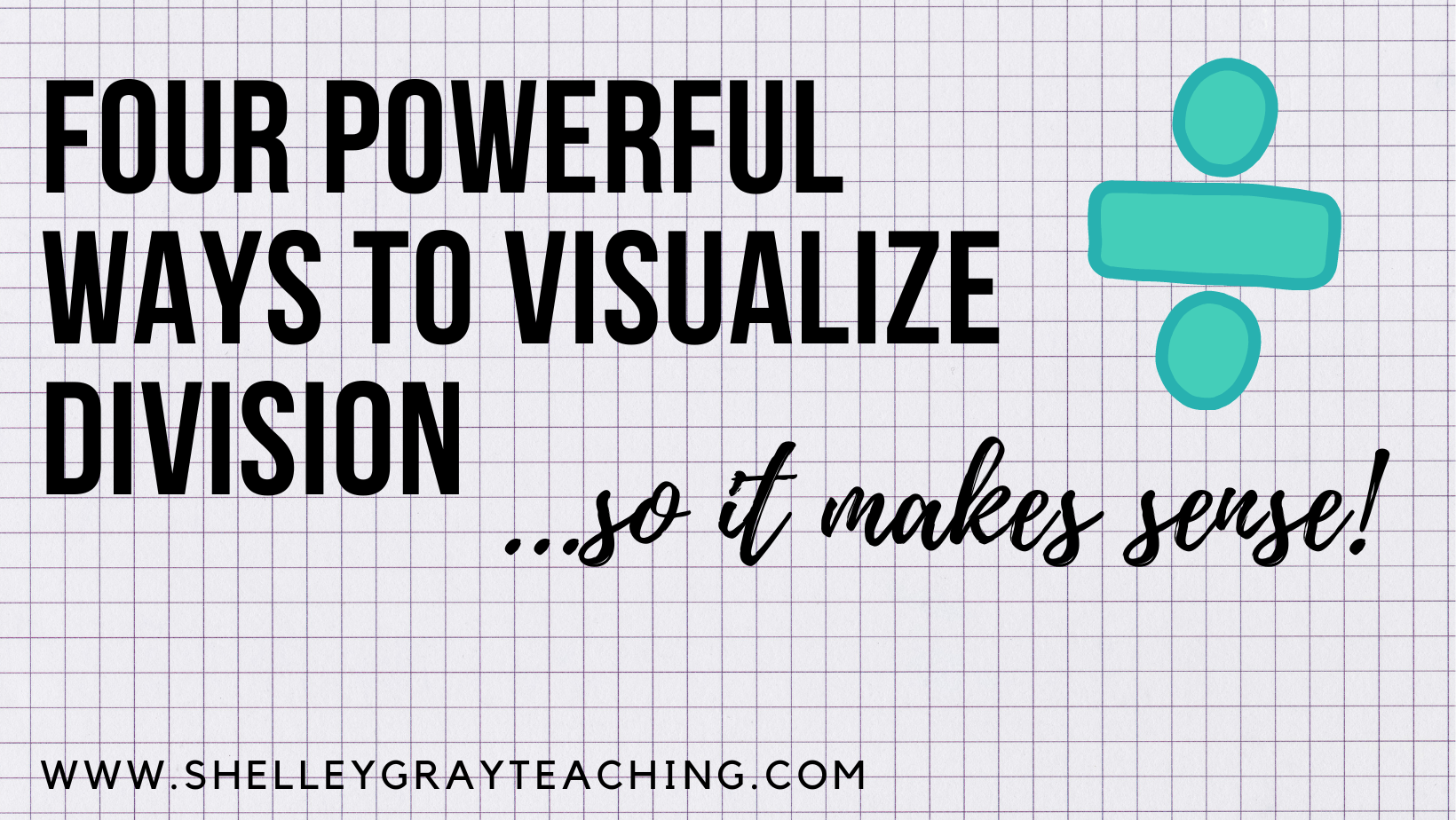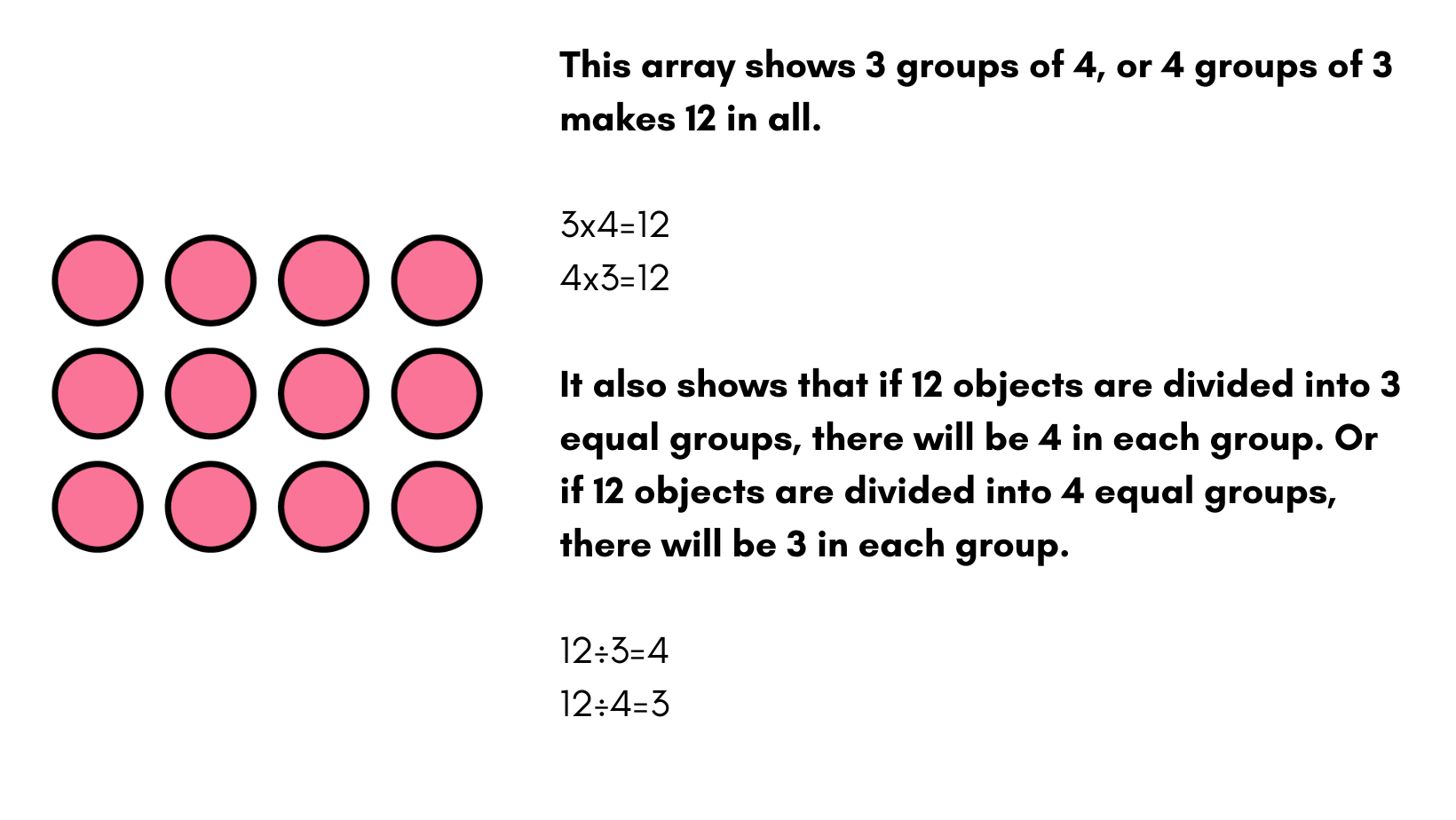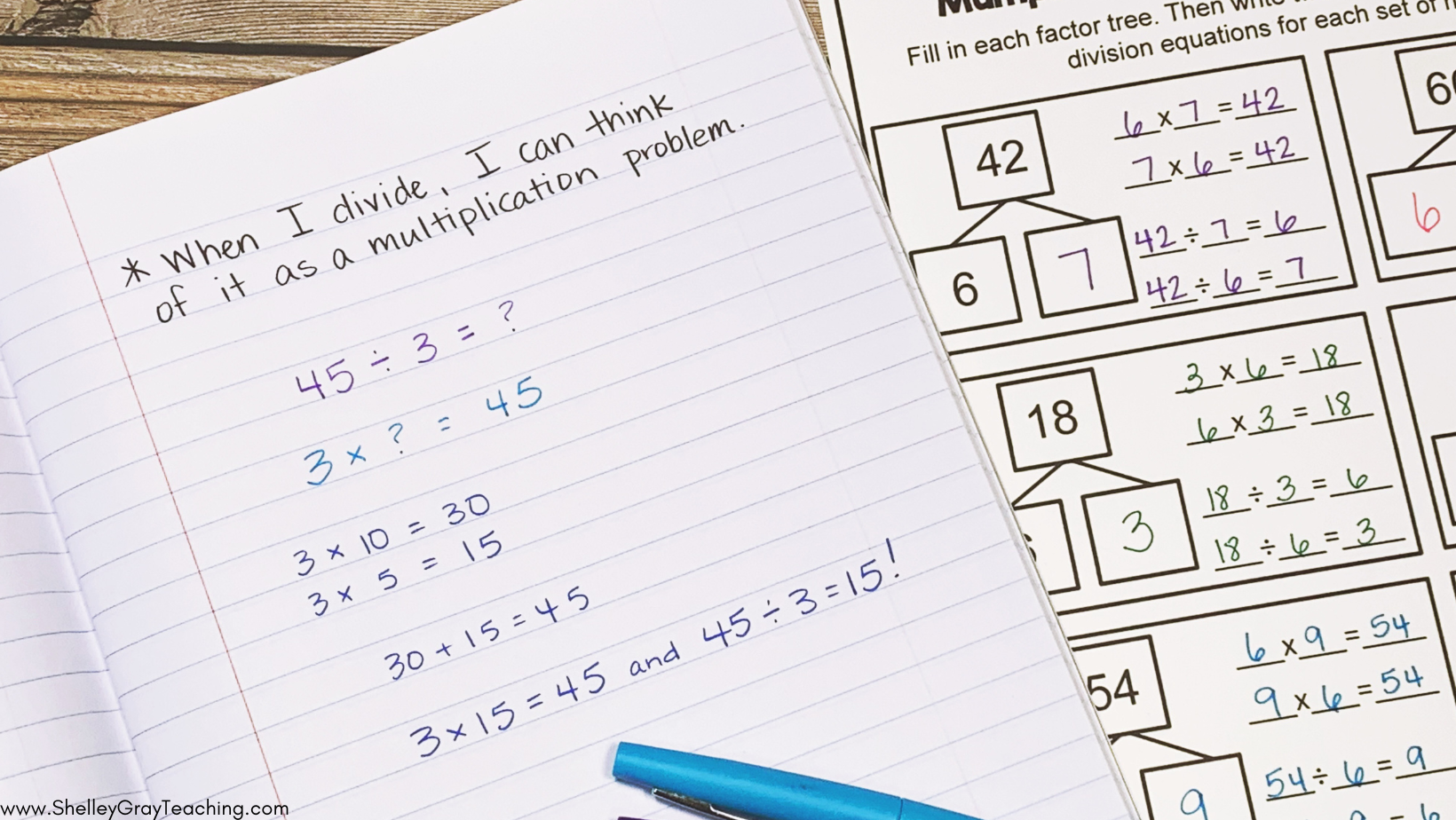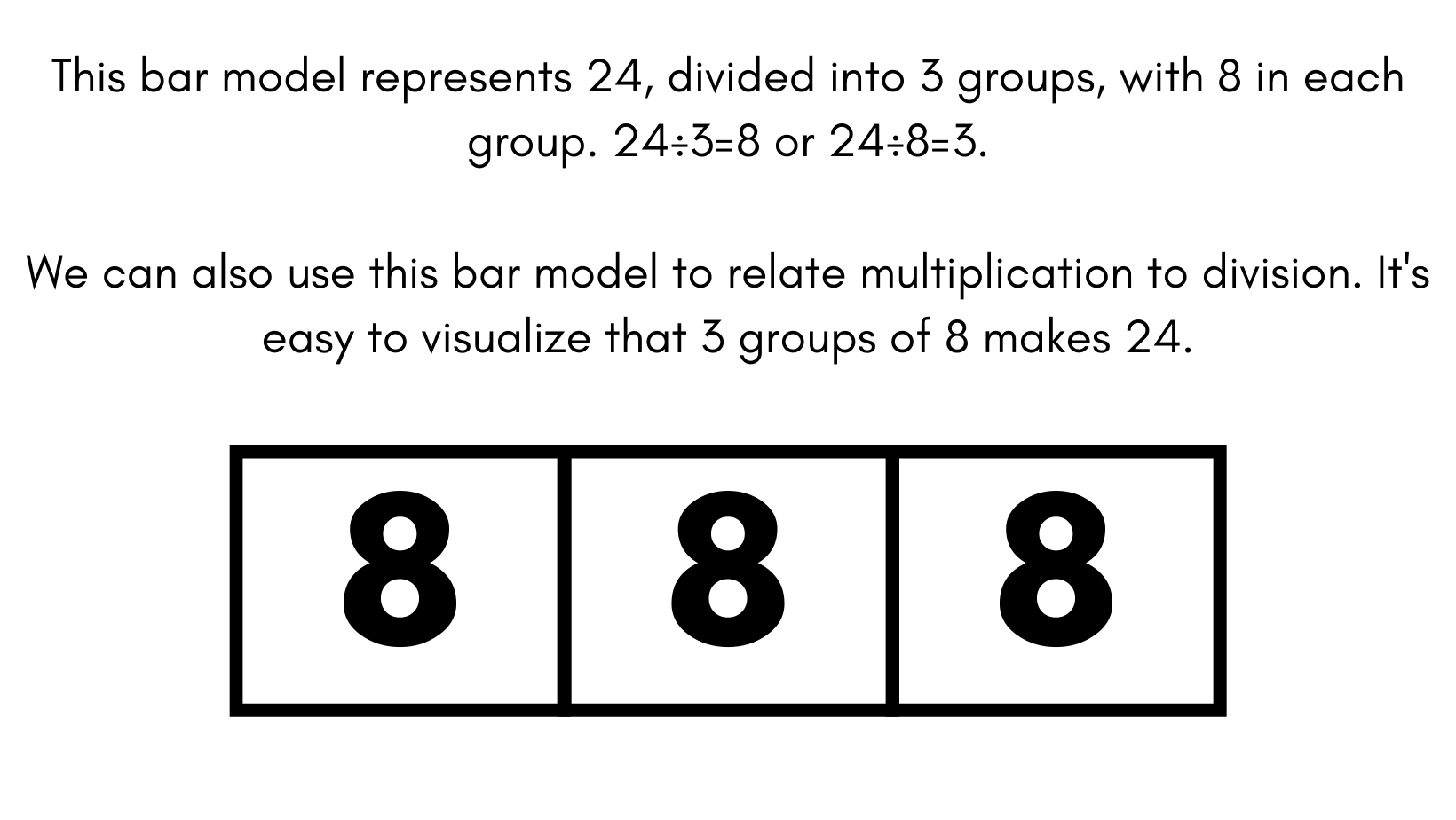# Four Powerful Ways to Visualize Division (so it makes sense)Understanding what division really means is essential to being able to divide accurately and efficiently. Whether you are teaching basic or multi-digit division, using visual models helps students understand it on a deeper level to build their conceptual understanding.

## Visualizing Division With Arrays

Arrays are an essential visual for both multiplication and division. When we teach multiplication, we can show students how an array represents a number of equal groups, and the product is the total number of objects in the array.

When we teach division, we can show students how an array represents a total amount of objects, and we are dividing them into equal groups, or groups of a certain number.Relate to Multiplication

Arrays can help students see how multiplying and dividing are closely related. Now, after using the arrays to visualize multiplication and division, we can relate it to fact families. This transitions to showing students how they can use multiplication to solve or check division problems.Find the activity sheets pictured above in The Division Station: a Self Paced Station for Division Fluency

## Equal Groups Using Manipulatives or Drawings

Making equal groups works well to model how a set of objects can be divided equally. As students work with larger numbers, they can use this same strategy – making equal groups with tens or hundreds instead of only using ones.## Bar Models for Division

Bar models can be used as a transition from more concrete, hands-on experiences to a more abstract representation. Bar models will continue to be useful as students progress to dividing decimals and fractions in older grades.## Division as Repeated Subtraction on a Number Line

Repeated subtraction shows the opposite of repeated addition as multiplication. We can use number lines to make this repeated subtraction more visual.

When students learn simple repeated subtraction, strategies such as partial quotients and the standard algorithm make more sense, as we have already built this understanding of how subtracting and dividing are related.

Related: Partial Quotients Strategy for Dividing Multi-Digit NumbersRemember, our main goal any operation is to build understanding, not build human calculators. Although speed and accuracy is still a goal, we have to build the understanding first.